# How the Compute Accuracy For Object Detection tool works

The Compute Accuracy For Object Detection tool calculates the accuracy of a deep learning model by comparing the detected objects from the Detect Objects Using Deep Learning tool to ground reference data. The accuracy of a model is evaluated using four accuracy metrics: the Average Precision (AP), the F1 score, the COCO mean Average Precision (mAP), and the Precision x Recall curve.

## Interpret model results

To understand the outputs from the Compute Accuracy For Object Detection tool, we must first have some understanding of detection model results.

In object detection and classification, a model can predict a positive class or a negative class, and the predictions can be true or false. For example, when detecting the presence of trees in an image, the positive class may be "Tree", while the negative class would be "No Tree". A true prediction occurs when the prediction is correct, and a false prediction occurs when the prediction is incorrect.

In the image below, the red bounding boxes indicate a positive prediction, where the model predicted that there is a tree present. The dark blue bounding boxes indicate a negative prediction, where the model predicted that there is no tree present.

The interpretation of each bounding box is explained in the table below.

NumberDescription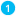True positive—The model predicted that there is a tree, and it is correct.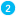False positive—The model predicted that there is a tree, and it is incorrect.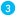False negative—The model predicted that there is no tree, and it is incorrect.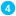True negative—The model predicted that there is no tree, and it is correct.

## Accuracy outputs

The accuracy of an object detection model depends on the quality and number of training samples, the input imagery, the model parameters, and the requirement threshold for accuracy.

The Intersection over Union (IoU) ratio is used as a threshold for determining whether a predicted outcome is a true positive or a false positive. IoU is the amount of overlap between the bounding box around a predicted object and the bounding box around the ground reference data.The intersecting area of the predicted bounding box and the ground reference bounding boxThe total area of the predicted bounding box and ground reference bounding box combined

The output accuracy table and accuracy report generated by the Compute Accuracy For Object Detection tool each contain a suite of accuracy metrics that depend on the IoU threshold and the performance of the model. The accuracy metrics are described below:

• Precision— Precision is the ratio of the number of true positives to the total number of positive predictions. For example, if the model detected 100 trees, and 90 were correct, the precision is 90 percent.
``Precision = (True Positive)/(True Positive + False Positive)``
• Recall—Recall is the ratio of the number of true positives to the total number of actual (relevant) objects. For example, if the model correctly detects 75 trees in an image, and there are actually 100 trees in the image, the recall is 75 percent.
``Recall = (True Positive)/(True Positive + False Negative)``
• F1 score—The F1 score is a weighted average of the precision and recall. Values range from 0 to 1, where 1 means highest accuracy.
``F1 score = (Precision × Recall)/[(Precision + Recall)/2]``
• Precision-recall curve—This is a plot of precision (y-axis) and recall (x-axis), and it serves as an evaluation of the performance of an object detection model. The model is considered a good predictive model if the precision stays high as the recall increases.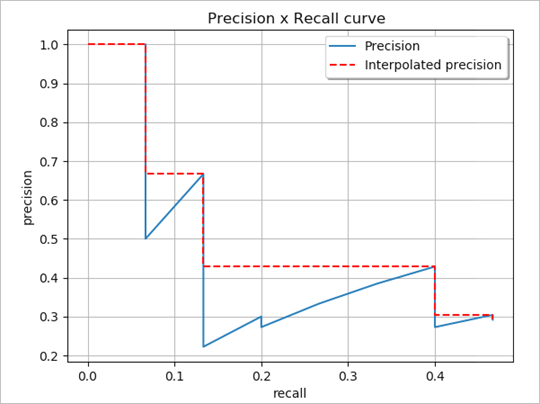The precision-recall curve (Padilla et al, 2020) is shown.
• Average Precision—Average Precision (AP) is the precision averages across all recall values between 0 and 1 at various IoU thresholds. By interpolating across all points, AP can be interpreted as the area under the curve of the precision-recall curve.
• Mean Average Precision—The Mean Average Precision (mAP) is the average AP over multiple IoU thresholds. For example, mAP@[0.5:0.05:0.95] corresponds to the AP for IoU ratio values ranging from 0.5 to 0.95, at intervals of 0.05, averaged over all classes.

## References

Hui, Jonathan. "mAP (mean Average Precision) for Object Detection." Posted March 6, 2018 on Medium. https://medium.com/@jonathan_hui/map-mean-average-precision-for-object-detection-45c121a31173.

Padilla, Rafael, Sergio L. Netto, and Eduardo A. B. da Silva. "A Survey on Performance Metrics for Object-Detection Algorithms." 2020 International Conference on Systems, Signals and Image Processing (IWSSIP), 237-242 (2000).

"Detection Evaluation." COCO Common Objects in Context, accessed October 15, 2020, https://cocodataset.org/#detection-eval.